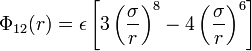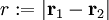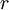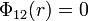# 8-6 Lennard-Jones potential

(diff) ← Older revision | Latest revision (diff) | Newer revision → (diff)$\Phi_{12}(r) = \epsilon \left[ 3\left(\frac{\sigma}{r} \right)^{8} - 4\left( \frac{\sigma}{r}\right)^6 \right]$
•$r := |\mathbf{r}_1 - \mathbf{r}_2|$
•$\Phi_{12}(r)$ is the intermolecular pair potential between two particles or sites
•$\sigma$ is the diameter (length), i.e. the value of$r$ at which$\Phi_{12}(r)=0$
•$\epsilon$ is the well depth (energy)